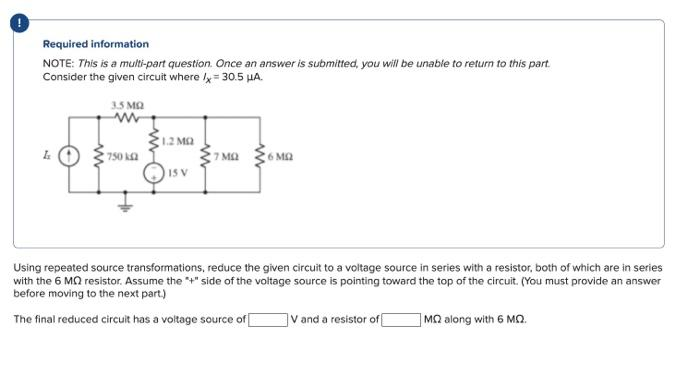# (Solved): Required information NOTE: This is a multi-part question. Once an answer is submitted, you will be u ...

Required information NOTE: This is a multi-part question. Once an answer is submitted, you will be unable to return to this part. Consider the given circuit where /x = 30.5 μA. Ix 3.5 ΜΩ 750 km2 1.2 ΜΩ 15 V · 7 ΜΩ 6 ΜΩ Using repeated source transformations, reduce the given circuit to a voltage source in series with a resistor, both of which are in series with the 6 MQ resistor. Assume the "+" side of the voltage source is pointing toward the top of the circuit. (You must provide an answer before moving to the next part.) The final reduced circuit has a voltage source of V and a resistor of ΜΩ along with 6 ΜΩ.Required information NOTE: This is a multi-part question. Once an answer is submitted, you will be unable to refurn to this part. Consider the given circuit where . Using repeated source transformations, reduce the given circuit to a voltage source in series with a resistor, both of which are in series with the resistor. Assume the "+" side of the voltage source is pointing toward the top of the circuit. (You must provide an answer before moving to the next part) The final reduced circuit has a voltage source of and a resistor of along with .

We have an Answer from Expert